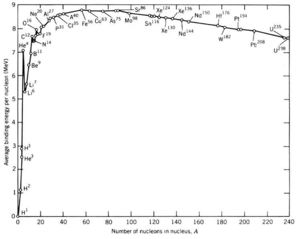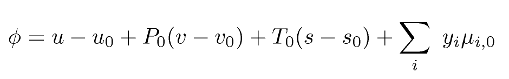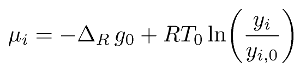The Exergy of Nuclear Fuels and Nuclear Radiation

Introduction to ExergyFig. 1: Nuclear binding energy of known nuclides. (Source: Wikimedia Commons)

When discussing the thermodynamics of energy systems, it is common to analyze the energy of a particular resource or energy flow of a particular process. Yet analyzing systems in this way, on the basis of energy alone, gives no indication of the quality of the energy being converted or transferred. For example, the gas molecules in the atmosphere have a great amount of energy. They have kinetic energy from translating and rotating, vibrational energy from fluctuations in bond lengths, and potentially even electronic energy from having electrons in excited states. While atmospheric gas molecules contain a lot of energy, this energy is of little value to one trying to design a work-producing energy system as all of the gas molecules are in equilibrium with each other; since all the molecules are at the same state, there is no pathway between two different states along which work could be extracted.

To better quantify the quality, or usefulness, of an energy resource, an exergy approach can be employed. The thermodynamic concept of exergy refers to the amount of useful work that can be extracted from a resource due to the fact that the resource is out of equilibrium with its environment. The resource or system could be at a different temperature or pressure than the environment, resulting in thermomechanical exergy, or it could have a different chemical composition than the environment, meaning it has chemical exergy. If none of these differences exist and the system is in complete equilibrium with its environment, than the system is considered to be at the dead state. 

To determine the amount of exergy a resource has, the first and second laws of thermodynamics can be combined to analyze a perfectly reversible process that goes from the resource state to the dead state. A perfectly reversible process (meaning the entropy generation term is set to 0) is chosen because it is the maximum useful work that is of interest. Combining the first and second laws in such a way results in the following equation for exergy: [1,2]In the above equation, φ is specific exergy (J/kg), u is specific internal energy (J/kg), P is pressure (Pa), v is specific volume (m3/kg), T is temperature (K), s is entropy(J/kg-K), and the subscript o denotes the dead state. The last term represents the chemical exergy of a resource, with i indicating a particular species component of the resource, yi indicating the mole fraction of that species, and μi indicating the chemical potential of that species. The chemical potential of a species is determined from the change in Gibbs free energy of its formation reaction from reference species and from the concentration of the species in the resource relative to the concentration of the species in the environment, as illustrated in the below equation.Nuclear Exergy

While thermomechanical and chemical exergy are well-defined parameters, there is no similar definition for nuclear exergy. Researchers have proposed several methods to define the exergy of nuclear fuels and of nuclear radiation, but have yet to reach a consensus. Furthermore, some of the assumptions used need to be revisited and updated.

Exergy of Nuclear Fuels

Eriksson, Islam and Skagerstam developed a model for the cosmic creation of nuclear exergy during the big bang.  They treated the nascent nucleon gas as a non-relativistic Maxwell-Boltzmann gas, and using the equilibrium and actual distribution of nucleons over four nuclear states (defined to be a free proton, free neutron, nucleon bound in He-4, or nucleon bound in Fe-56), they developed expressions for the exergy of a nucleon. They concluded that once the Universe had cooled to a temperature of 109 K, nucleons each had an exergy of 7.72 MeV. Furthermore, they determined that nuclear exergy creation started approximately 10 seconds after the big bang, 85% of nuclear exergy was created in the first hour, and effectively 100% of nuclear exergy was created within 24 hours.

Several works which have performed exergy analyses of nuclear power plants have defined the exergy of a nuclear fuel to be approximately equal to the energy equivalent of the rest mass change of a reaction. [4-6] They justify this definition by saying that the temperatures at which nuclear reactions occur are so high that the energy released during them is of high enough quality that all of it can be reclaimed as useful work. In a similar manner, Hermann defined nuclear exergy based on the binding energy of a nucleus, or the energy equivalent of the difference in rest mass between a fuel and its decay products.  With this approach, he evaluated the exergy of fuels that could be used in currently proposed fission and fusion cycles, namely U-235, U-238, and Th-232 on the fission side and H-2, Li-6, and Li-7 on the fusion side. With this approach, he found that uranium has an exergy of 77 TJ/kg, resulting in a worldwide exergy reservoir of 1 YJ (1024 J) from uranium ore and 350 YJ from seawater uranium; thorium has an exergy of 78 TJ/kg, resulting in a worldwide exergy reservoir of 300 ZJ; lithium (which can breed tritium for fusion) has an exergy of 226 TJ/kg for a worldwide exergy reservoir of 3.1 YJ; and finally, naturally-occurring deuterium in the ocean has an exergy of 345 TJ/kg, for an astounding worldwide exergy reservoir of 106 YJ.

One of the problems with the above approaches is the failure to define an appropriate dead state. Of the preceding works, only Hermann addresses this issue.  He does so by assuming that the products of currently proposed nuclear cycles have zero exergy. This is not an accurate depiction, however, as the products of these reactions can still undergo further fission or fusion and subsequently release more energy which could be converted to useful work. Hermann's approach assumes that the entirety of a fuel's exergy is captured in a single decay reaction and implies that no more work can be extracted after such a reaction, neglecting all of the work potential left in the decay products and by extension in nuclear waste. A more appropriate choice for the nuclear dead state would be Ni-62, which is known to be the most stable nuclide as it has the lowest specific bound energy of any known nuclide (or highest negative specific bound energy, as shown in Fig. 1). 

Exergy of Nuclear Radiation

Badescu and Isvornu used both a classical statistical thermodynamic approach and a quantum statistical thermodynamic approach to determine the exergy of nuclear radiation. [8,9] In the classical approach, emitted particles are treated as distinguishable and therefore makes use of Boltzmann statistics, while in the quantum approach, emitted particles are treated as indistinguishable and therefore Fermi statistic are used instead. In both cases, the exergy (or exergy flux) due to nuclear radiation is equal to the internal energy (or internal energy flux) of the emitted particle times an efficiency factor. For exergy, the efficiency factor is 1 - To/T in the classical approach and 1 - a To/T - b (To/T)5/2 (where a and b are constants) in the quantum approach. With exergy flux, the efficiency factor is again 1 - To/T in the classical approach, but it is 1 - c To/T - d (To/T)3 (where c and d are constants) in the quantum approach. These results are similar to those obtained for the exergy of thermal radiation. In this work, the dead state of a particle was taken to be the particle at the temperature and pressure of the surrounding environment.

Conclusion

While there is still work to be done on nuclear exergy, particularly with the definition of the exergy of nuclear fuels and their associated dead state, all works agree that the energy available from nuclear resources is tremendous. Nuclear energy should therefore be strongly considered as a viable energy resource for the world moving forward, especially given the limited supply of fossil fuels and the fact that nuclear power plants provide CO2-free electricity.

© Carol Regalbuto. The author grants permission to copy, distribute and display this work in unaltered form, with attribution to the author, for noncommercial purposes only. All other rights, including commercial rights, are reserved to the author.

References

 Y. Cengel and M. Boles. Thermodynamics: An Engineering Approach, 7th Ed. (McGraw-Hill, 2010).

 W. A. Hermann, "Quantifying Global Exergy Resources," J. Energy 31 1685 (2006).

 K.-E. Eriksson, S. Islam, B.-S. Skagerstam, "A Model for the Cosmic Creation of Nuclear Exergy," Nature 296 540 (1982).

 W. R. Dunbar, S. D Moody,and N. Lior, "Exergy Analysis of an Operating Boiling-Water Reactor Nuclear Power Station," J. Energy Convers. Mgmt. 36, 149 (1995).

 A. Durmayaz and H. Yavuz, "Exergy Analysis of a Pressurized-Water Reaction Nuclear Power Plant," J. Appl. Energy 69 39 (2001).

 M. A. Rosen, "Energy- and Exergy-Based Comparison of Coal-Fired and Nuclear Steam Power Plants," Exergy Int. J. 1, 180 (2001).

 G. Audi, A. H. Wapstra, C. Thibault, "The AME2003 Atomic Mass Evaluation, Part II: Tables, Graphs, and References," Nucl. Physics A 729 337 (2003).

 V. Badescu and D. Isvoranu, "Classical Statistical Thermodynamics Approach for the Exergy of Nuclear Radiation," Europhys. Lett. 80, 30003 (2007).

 V. Badescu, "Exergy of Nuclear Radiation - a Quantum Statistical Thermodynamics Approach," Cent. Eur. J. Phys. 7 141 (2009).